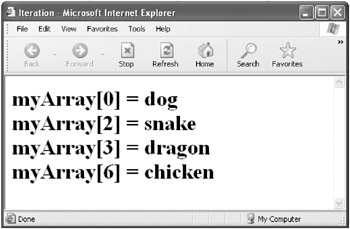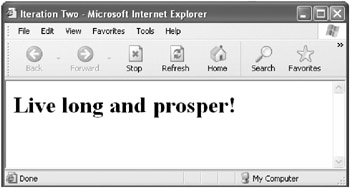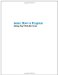# Iterating Through an Array

Probably the most important thing you can do to or with an array is iterate through it. You could say that “Arrays live to be iterated!”

One could also say “Don’t procrastinate, iterate!” But let’s move from sayings and slogans toward understanding: What does iterate mean?

To iterate through an array means to loop (or cycle) through the array, checking and/or doing something with each and every element.

The easiest and most common way to iterate is to use a variable that represents the index of the array in a for loop. You also need to know how many elements are in an array, and you can determine this using the length property of the array.

If you know that the range of the index of your array is contiguous and begins at element zero, then iterating through the array is easy. Here’s the general pattern:

If you have an array created like this:

` var theArray = new Array(10); `

then the index values for theArray range from one to nine. (You don’t really need to know the upper bound of the index of the array, but it’s good to keep in mind that the array index starts at zero.)

The following statement can be used to iterate through theArray:

` for (var i = 0; i < theArray.length; i++) {     // do something with theArray[i]  } `

This for statement starts with zero, increments by one each time it goes through the for loop (as directed by the i++ clause in the statement), and terminates when the index becomes equal to the value of the array length property. (In the example, the loop terminates after an index value of 9 has been processed.) If you need a refresher on how for loops work, please refer to Chapter 4, “ Working with Loops.”

As I mentioned earlier, JavaScript arrays aren’t necessarily contiguous, another way of saying that they’re sparse. This means that if you’re iterating through a sparse array, you need to check each element within the loop to make sure it’s defined.

For example, suppose you have an array defined as follows:

` var myArray = new Array(4);  myArray = "dog";  myArray = "cat";  myArray = "snake";  myArray = "dragon";  myArray = "chicken";  myArray = undefined; `

In this scenario, elements 0, 2, 3, and 6 of the array are defined; elements 1, 4, and 5 are undefined. Let’s suppose you’d like to display the index for each defined element of the array along with its value, as shown in Figure 6-2.Figure 6-2: The defined elements of the sparse array

You can use the normal pattern of statements to loop through the array:

` for (var i = 0; i < myArray.length; i++){  } `

Inside the for loop, you’d normally use a statement like this:

` document.write("myArray[" + i + "] = " + myArray[i] + "<br>"); `

to display the array index and values. Here’s the loop code so far:

` for (var i = 0; i < myArray.length; i++){      document.write("myArray[" + i + "] = " + myArray[i] + "<br>");  } `

If the code runs as it stands, you’ll see the undefined values (see Figure 6-3).Figure 6-3: The undefined values of the array

The way to fix this is to add a conditional statement that checks to make sure that each array element is defined before displaying it. Here’s what the loop, modified to check for undefined array values, looks like:

` for (var i = 0; i < myArray.length; i++){     if (myArray[i] != undefined)        document.write("myArray[" + i + "] = " + myArray[i] + "<br>");  } `

Listing 6-2 shows an HTML page that displays only the defined elements of myArray.

Listing 6.2: Iterating Through a Sparse Array` <HTML> <HEAD> <TITLE>     Iteration     </TITLE> </HEAD> <BODY> <H1> <SCRIPT>        var myArray = new Array(4);        myArray = "dog";        myArray = "cat";        myArray = "snake";        myArray = "dragon";        myArray = "chicken";        myArray = undefined;       for (var i = 0; i < myArray.length; i++){         if (myArray[i] != undefined)          document.write("myArray[" + i + "] = " + myArray[i] + "<br>");       }     </SCRIPT> </H1> </BODY> </HTML> `By the way, you can use Boolean evaluation as another way to determine whether an array element is defined.

 Note There’s nothing wrong with the test if (myArray[i] != undefined) to determine if an array element is undefined; but there are others way to achieve the same result. (As I explained in Chapter 2, “ Understanding Types, Variables, and Statements,” != is the comparison operator that means inequality.)

The expression myArray[i] evaluates to true if the array element is defined and false if it’s undefined. So, you could replace the iteration code shown in Listing 6-2 with the following loop:

` for (var i = 0; i < myArray.length; i++){     if (myArray[i])        document.write("myArray[" + i + "] = " + myArray[i] + "<br>");      } `

This works just fine most of the time except in the situation in which you’ve stored Boolean values in the array elements. Using if (myArray[i]) as the test, the element myArray = true evaluates to true, but the element myArray = false evaluates to false, which isn’t what you’d like from the viewpoint of deciding whether the element is defined (it’s defined, but with a Boolean value of false). As long as you’re not storing Boolean values in your array, you can use Boolean evaluation as a simple test for whether the array element is defined.Using the Array Length Property

The length property of an array is an integral number that’s one larger than the largest index of a defined element of the array. If you think about this, it means that the length property isn’t always equal to the number of elements in an array because JavaScript arrays can be noncontiguous and sparsely populated.

You should also know that an array’s length property can be used to write (as well as to read) the length of an array. If you set the value of the length property greater than its current value, new undefined elements are added at the end of the array to extend it to the newly designated size.

If you set the length property to a value that’s smaller than its current value, the array is truncated to its new length. Elements with an index value greater than the new length are discarded and their values are gone forever.

Truncating an array by setting its length property to a smaller than current value is the only way to actually remove elements from an array (as opposed to deleting the elements, which makes them undefined and doesn’t impact the length of the array).### Seeing Another Array Iteration Example in Action

Let’s go ahead and work through another example of iterating through an array. This time, we’ll break up the tasks using functions (for more information about functions, see Chapter 5, “ Understanding Functions ”).

There are three tasks to perform:

1. Create a function to create and populate an array.

2. Create another function to generate a text string based on the values stored in the array elements.

3. Display the text string.

Let’s take these steps from the top.

To Create and Populate an Array:

1. Create the declaration for the function makeArray that will be used to create and populate the array:

` function makeArray() {  } `

Note that there are no arguments passed to this function.

2. Use the new operator to construct a new array with four elements:

` var myArray = new Array(4); `

3. Assign values to the elements of the array:

` myArray = "Live";  myArray = "long";  myArray = "and";  myArray = "prosper!"; `

4. Send the populated array back to the code that called the makeArray function as the return value of the function:

` return myArray; `

Here’s the complete makeArray function:

` function makeArray() {      var myArray = new Array(4);      myArray = "Live";      myArray = "long";      myArray = "and";      myArray = "prosper!";      return myArray;  } `

Next, let’s create a function named showArray that iterates through the elements of the passed array to generate a single text string based on the values of the array elements separated by spaces. (Note that the code assumes that the index values of the array elements are contiguous.)

To Generate a Text String by Iterating Through Array Elements:

1. Create a declaration for the showArray function that takes a single argument (the code within the function assumes the argument to be an array):

` function showArray(theArray){  } `

2. Declare a variable to hold the text string that will be returned:

` var quote = ""; `

3. Iterate through the array elements:

` for (var i = 0; i < theArray.length; i++){  } `

4. For each iteration, append the value of the array element and a single space to the return string using the concatenation operator:

` for (var i = 0; i < theArray.length; i++){     quote += theArray[i] + " ";  } `

5. Outside, and beneath, the for loop, return the fully generated text string:

` return quote; `

Here’s the complete code for the showArray function:

` function showArray(theArray){       var quote = "";       for (var i = 0; i < theArray.length; i++){        quote += theArray[i] + " ";        }     return quote;  } `

The final task is to display the generated text string.

You can easily do this from within the body of an HTML document by calling each of the two functions, makeArray and showArray, in order.

To Display the Generated Text:

1. Within the body of an HTML document, declare a variable x and assign to it the return value of the makeArray function:

` var x = makeArray(); `

2. Use the document.write method to display the return value of the showArray function when it has been passed the array stored as the value of x:

` document.write(showArray(x)); `

The actual program in the body of this HTML page consists of only two statements:

` var x = makeArray();  document.write(showArray(x)); `

These two statements appear to be simple, which is part of the idea. The actual work of making the array goes on in the function makeArray, just as the actual work of showing the array is performed by the function showArray. You don’t necessarily need to know exactly how makeArray and showArray do their dastardly deeds to use them. As you’ll see in Chapter 7, “ Working with Objects,” this programmatic separation, and opacity, is at the heart of encapsulation, one of the key concepts of object-oriented programming.

Listing 6-3 shows the complete code for creating, populating, and displaying the values in an array in an HTML page. If you open this page in a Web browser, it should look like Figure 6-4.

Listing 6.3: Using Functions to Iterate Through an Array` <HTML> <HEAD> <TITLE>     Iteration Two     </TITLE> <SCRIPT>     function makeArray() {         var myArray = new Array(4);         myArray = "Live";         myArray = "long";         myArray = "and";         myArray = "prosper!";         return myArray;     }      function showArray(theArray){        var quote = "";        for (var i = 0; i < theArray.length; i++){           quote += theArray[i] + " ";        }        return quote;      }     </SCRIPT> </HEAD> <BODY> <H1> <SCRIPT>        var x = makeArray();        document.write(showArray(x));     </SCRIPT> </H1> </BODY> </HTML> `Figure 6-4: The value of the elements of an array are displayed as a text string.

The process shown in this example may seem a little convoluted to you, but it’s worth following to make sure you understand it. By breaking up the task into three parts, each of the parts has become effectively independent.

The showArray function is completely independent from the function that creates and populates the array. The showArray function is also independent from the code that displays the text string. The three parts of this program even use a different variable name for the array involved (myArray, theArray, and x).

The only connection between the three parts of the code is that the two statements in the body of the HTML document calls each of the functions in turn.

Can you modify this example to display a saying of your own, perhaps adding line breaks to the text display?Learn How to Program Using Any Web Browser
ISBN: 1590591135
EAN: 2147483647
Year: 2006
Pages: 115
Authors: Harold Davis

Similar book on Amazon